Equations Involving Logarithms and Exponentials

Recall that if, then.

(Notice thatis undefined if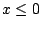.)

We will denote natural logarithms (logarithms with base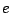) by.

Furthermore,.

Ex 1 Solve the equation.

Sol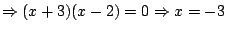or. Sincedoes not check in the original equation,is the only solution.

Ex 2 Solve the equation, and write your answer using natural logarithms.

Sol Dividing by 6 gives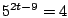, and then taking natural logarithms of both sides gives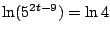or. Then, soand therefore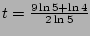. Since, we can also write the answer as.

Pr A Solve the equation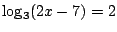.

Pr B Solve the equation, writing your answer using natural logarithms.

Pr C Find all solutions of.

Pr D Solve the equation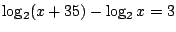.

Pr E Find all solutions of.

Pr 1 Solve the equation.

Pr 2 Solve the equation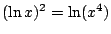.

Pr 3 Solve the equation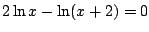.

Pr 4 Solve the equation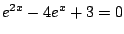.

Pr 5 Solve the equation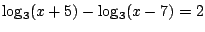.

Pr 6 Solve the equation.

Pr 7 Solve the equation, and write your answer using natural logarithms.